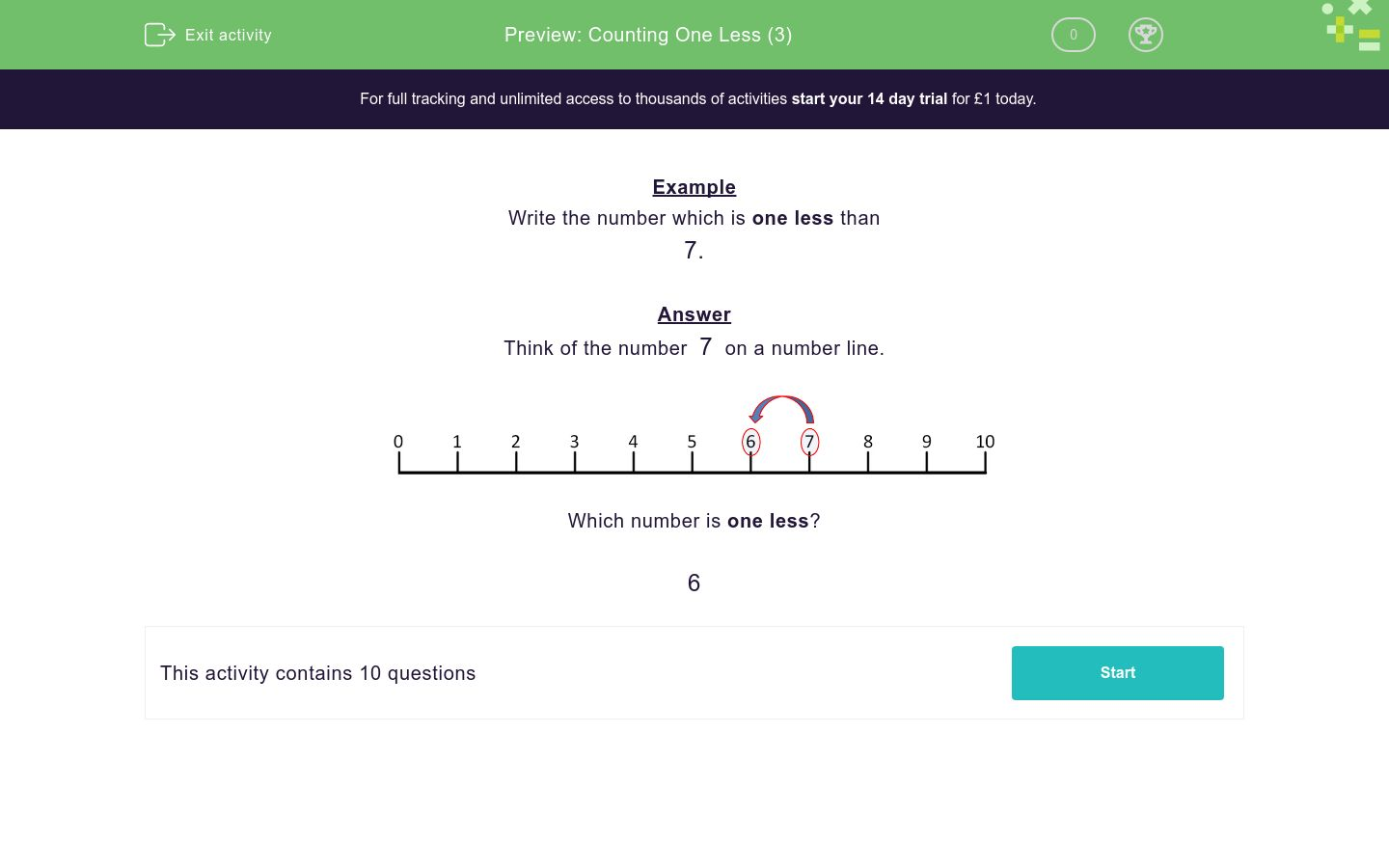# Counting One Less (3)

In this worksheet, children must write the number that is one less than the number shown.Key stage:  KS 1

Curriculum topic:   Number: Number and Place Value

Curriculum subtopic:   Identify One More/One Less

Difficulty level:### QUESTION 1 of 10

Example

Write the number which is one less than

7.

Think of the number  7  on a number line.Which number is one less?

6

Write the number which is one less than

4

Write the number which is one less than

5

Write the number which is one less than

2

Write the number which is one less than

7

Write the number which is one less than

3

Write the number which is one less than

1

Write the number which is one less than

6

Write the number which is one less than

8

Write the number which is one less than

9

Write the number which is one less than

10

• Question 1

Write the number which is one less than

4

3
EDDIE SAYS
0... 1... 2... 3... 4
• Question 2

Write the number which is one less than

5

4
EDDIE SAYS
0... 1... 2... 3... 4... 5
• Question 3

Write the number which is one less than

2

1
EDDIE SAYS
0... 1... 2
• Question 4

Write the number which is one less than

7

6
EDDIE SAYS
0... 1... 2... 3... 4... 5... 6... 7
• Question 5

Write the number which is one less than

3

2
EDDIE SAYS
0... 1... 2... 3
• Question 6

Write the number which is one less than

1

0
EDDIE SAYS
0... 1
• Question 7

Write the number which is one less than

6

5
EDDIE SAYS
0... 1... 2... 3... 4... 5... 6
• Question 8

Write the number which is one less than

8

7
EDDIE SAYS
0... 1... 2... 3... 4... 5... 6... 7... 8
• Question 9

Write the number which is one less than

9

8
EDDIE SAYS
0... 1... 2... 3... 4... 5... 6... 7... 8... 9
• Question 10

Write the number which is one less than

10

9
EDDIE SAYS
0... 1... 2... 3... 4... 5... 6... 7... 8... 9... 10
---- OR ----

Sign up for a £1 trial so you can track and measure your child's progress on this activity.

### What is EdPlace?

We're your National Curriculum aligned online education content provider helping each child succeed in English, maths and science from year 1 to GCSE. With an EdPlace account you’ll be able to track and measure progress, helping each child achieve their best. We build confidence and attainment by personalising each child’s learning at a level that suits them.

Get started